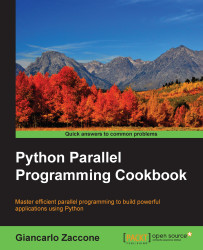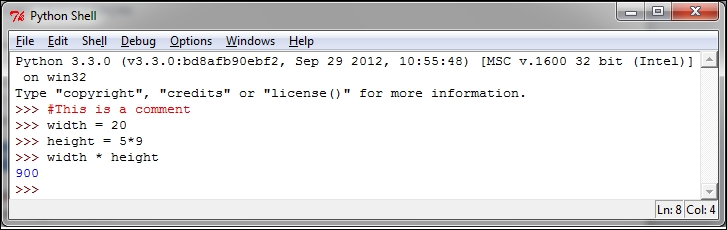•#### Python Parallel Programming Cookbook#### Overview of this book

This book will teach you parallel programming techniques using examples in Python and will help you explore the many ways in which you can write code that allows more than one process to happen at once. Starting with introducing you to the world of parallel computing, it moves on to cover the fundamentals in Python. This is followed by exploring the thread-based parallelism model using the Python threading module by synchronizing threads and using locks, mutex, semaphores queues, GIL, and the thread pool. Next you will be taught about process-based parallelism where you will synchronize processes using message passing along with learning about the performance of MPI Python Modules. You will then go on to learn the asynchronous parallel programming model using the Python asyncio module along with handling exceptions. Moving on, you will discover distributed computing with Python, and learn how to install a broker, use Celery Python Module, and create a worker. You will understand anche Pycsp, the Scoop framework, and disk modules in Python. Further on, you will learnGPU programming withPython using the PyCUDA module along with evaluating performance limitations.
Python Parallel Programming CookbookCreditswww.PacktPub.comPrefaceFree Chapter
Getting Started with Parallel Computing and PythonProcess-based ParallelismAsynchronous ProgrammingDistributed PythonGPU Programming with PythonIndex## Introducing Python

Python is a powerful, dynamic, and interpreted programming language that is used in a wide variety of applications. Some of its features include:

• A clear and readable syntax

• A very extensive standard library, where through additional software modules, we can add data types, functions, and objects

• Easy-to-learn rapid development and debugging; the development of Python code in Python can be up to 10 times faster than the C/C++ code

• Exception-based error handling

• A strong introspection functionality

• Richness of documentation and software community

Python can be seen as a glue language. Using Python, better applications can be developed because different kinds of programmers can work together on a project. For example, when building a scientific application, C/C++ programmers can implement efficient numerical algorithms, while scientists on the same project can write Python programs that test and use those algorithms. Scientists don't have to learn a low-level programming language and a C/C++ programmer doesn't need to understand the science involved.

### Note

Although you can create Python programs with Notepad or TextEdit, you'll notice that it's much easier to read and write code using an Integrated Development Environment (IDE).

There are many IDEs that are designated specifically for Python, including IDLE (http://www.python.org/idle), PyCharm (https://www.jetbrains.com/pycharm/), and Sublime Text, (http://www.sublimetext.com/).

### How to do it…

Let's take a look at some examples of the very basic code to get an idea of the features of Python. Remember that the symbol `>>>` denotes the Python shell:

• Operations with integers:

```>>> # This is a comment
>>> width = 20
>>> height = 5*9
>>> width * height
900
```

Only for this first example, we will see how the code appears in the Python shell:Let's see the other basic examples:

• Complex numbers:

```>>> a=1.5+0.5j
>>> a.real
1.5
>>> a.imag
0.5
>>> abs(a)  # sqrt(a.real**2 + a.imag**2)
5.0
```
• Strings manipulation:

```>>> word = 'Help' + 'A'
>>> word
'HelpA'
>>> word
'A'
>>> word[0:2]
'He'
>>> word[-1]     # The last character
'A'
```
• Defining lists:

```>>> a = ['spam', 'eggs', 100, 1234]
>>> a
'spam'
>>> a
1234
>>> a[-2]
100
>>> a[1:-1]
['eggs', 100]
>>> len(a)
4
```
• The `while` loop:

```# Fibonacci series:
>>> while b < 10:
...       print b
...       a, b = b, a+b
...
1
1
2
3
5
8
```
• The `if` command:

First we use the `input()` statement to insert an integer:

```>>>x = int(input("Please enter an integer here: "))
```

Then we implement the `if` condition on the number inserted:

```>>>if x < 0:
...      print ('the number is negative')
...elif x == 0:
...      print ('the number is zero')
...elif x == 1:
...      print ('the number is one')
...else:
...      print ('More')
...
```
• The `for` loop:

```>>> # Measure some strings:
... a = ['cat', 'window', 'defenestrate']
>>> for x in a:
...     print (x, len(x))
...
cat 3
window 6
defenestrate 12
```
• Defining functions:

```>>> def fib(n):    # write Fibonacci series up to n
...     """Print a Fibonacci series up to n."""
...     a, b = 0, 1
...     while b < n:
...         print (b),
...         a, b = b, a+b
...
>>> # Now call the function we just defined:
... fib(2000)
1 1 2 3 5 8 13 21 34 55 89 144 233 377 610 987 1597
```
• Importing modules:

```>>> import math
>>> math.sin(1)
0.8414709848078965

>>> from math import *
>>> log(1)
0.0
```
• Defining classes:

```>>> class Complex:
...     def __init__(self, realpart, imagpart):
...         self.r = realpart
...         self.i = imagpart
...
>>> x = Complex(3.0, -4.5)
>>> x.r, x.i
(3.0, -4.5)
```